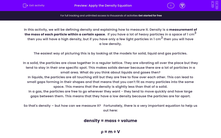# Apply the Density Equation

In this worksheet, students will calculate the density of irregular objects. They will also learn how to manipulate equations to solve problems.Key stage:  KS 4

Year:  GCSE

GCSE Boards:   AQA, AQA Trilogy, AQA Synergy, OCR 21st Century, OCR Gateway, Pearson Edexcel, Eduqas,

Popular topics:   Physics worksheets

Difficulty level:#### Worksheet Overview

In this activity, we will be defining density and explaining how to measure it. Density is a measurement of the mass of each particle within a certain space.  If you have a lot of heavy particles in a space of 1 cm3 then you will have a high density, but if you have only a few light particles in 1 cm3 then you will have a low density.

The easiest way of picturing this is by looking at the models for solid, liquid and gas particles.

In a solid, the particles are close together in a regular lattice. They are vibrating all over the place but they tend to stay in their one specific spot. This makes solids denser because there are a lot of particles in a small area. What do you think about liquids and gases then?

In liquids, the particles are all touching still but they are free to flow over each other. This can lead to small gaps forming in their shapes and that means that you can’t fit as many particles into the same space. This means that the density is slightly less than that of a solid.

In a gas, the particles are free to go wherever they want - they tend to move quickly and have large gaps between them. This means that they have a low density because the particles are far apart.

So that's density – but how can we measure it?   Fortunately, there is a very important equation to help us out here:

density = mass ÷ volume

ρ = m ÷ V

The sign ρ is the Greek letter rho – in this case it stands for density (because d was already used…), m is obviously mass and V is volume (as in how much space it takes up, not how loud it is…).

Mass is measured in kg, volume is measured in m3  and density is measured in kg/m3. The unit of measurement for density is just the unit of measurement for mass divided by the unit of measurement for volume. That is because the slash means divided by, so all we are saying is that the unit is kg divided by metres cubed.

If you know the unit of measurement of the thing you are trying to find, you can normally work out what needs to go into the equation to find the answer. For example, if you are asked to work out a speed in m/s, then you know that the equation you need is m divided by s.

Now let’s look at how to find the density of an irregular object.

Let’s say you have a rock – how will you work out the density of that object using this equipment?

[insert Royalty-free stock vector ID: 1362981782]

What do we need to know in order to calculate the density? It’s the mass and volume, right? So mass is nice and easy, you just put the rock on the balance and hey presto – there is your mass. For volume, it would be easy if it was a regularly shaped object, but it’s not. This is a problem that was solved by Archimedes back in the Ancient Greek times. He sat in the bath and saw the water spill out over the side – what he realised was that the amount of water that came out of the bath was the same as his volume – so all we need to do is make a fancy bath that will collect the water as it spills out.

This is an Archimedes or eureka can – and it measures the water displaced by an irregular object. In this case, we put the rock in and measure the amount of water in millilitres (ml) that comes out. 1 ml = 1 cm3.

All you need to do then is take your measurements and divide the mass by the volume and you’ve got your density!

One important point though - this only works for objects that sink!

Are you ready to tackle some questions now?

### What is EdPlace?

We're your National Curriculum aligned online education content provider helping each child succeed in English, maths and science from year 1 to GCSE. With an EdPlace account you’ll be able to track and measure progress, helping each child achieve their best. We build confidence and attainment by personalising each child’s learning at a level that suits them.

Get started